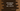# C sharp program to check if a number is positive, negative or zero# Check if a number is positive or negative in C# :

In this C# tutorial, we will learn how to check if a number is positive, negative or zero. The program will ask the user to enter a number. It will check if it is positive, negative or zero and finally print out the result.

With this tutorial, you will learn how to compare a number using if-else condition, how to take user input and how to write a message to the user.

## C# program :

``````using System;
namespace dotnet_sample
{
class Program
{
static void Main(string[] args)
{
//1
int n;
//2
Console.WriteLine("Enter a number : ");
//3
//4
if(n == 0)
{
Console.WriteLine(n + " is zero.");
}
else if(n > 0)
{
//5
Console.WriteLine(n + " is a positive number.");
}
else
{
//6
Console.WriteLine(n + " is a negative number.");
}
}
}
}``````

### Explanation :

The commented numbers in the above program denote the step numbers below :

1. Create one integer n to read the user input.
2. Ask the user to enter a number.
3. Read the number and store it in_ ‘n’._
4. Check if the value of_ ‘n’_ is 0 or not. If yes, print out that it is zero.
5. Else check if it is greater than 0 or not. If yes, print out that it is a positive number.
6. Else print that it is a negative number.

### Sample Output :

``````Enter a number :
0
0 is zero.

Enter a number :
-23
-23 is a negative number.

Enter a number :
45
45 is a positive number.``````

This program is available on Github